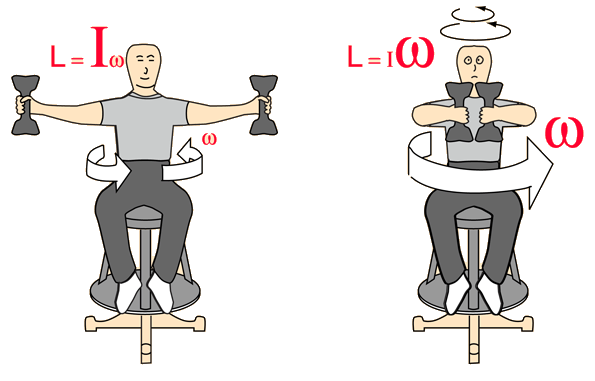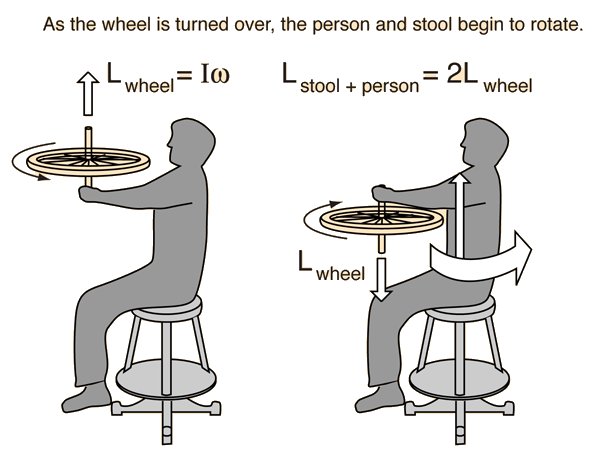# Rotating Stool

 The moment of inertia is large with the masses held out. For a given angular momentum, the angular velocity is relatively low. If the masses are pulled in, the moment of inertia is considerably decreased. Conservation of angular momentum dictates that the angular velocity must increase.Classroom Demonstration Video Example
Index

Vector rotation examples

 HyperPhysics***** Mechanics ***** Rotation R Nave
Go Back

# Rotating Stool

 Begin with the wheel rotating so that its angular momentum is upward. The man and the stool are at rest. The stool is on a bearing mount so that it can freely rotate. If the wheel is turned over, its angular momentum is now downward. But since there is nothing to exert a significant torque on the system, the angular momentum must have the original value upward. The man and the stool begin to rotate with angular momentum twice that of the wheel.Video Example
Index

Vector rotation examples

 HyperPhysics***** Mechanics ***** Rotation R Nave
Go Back